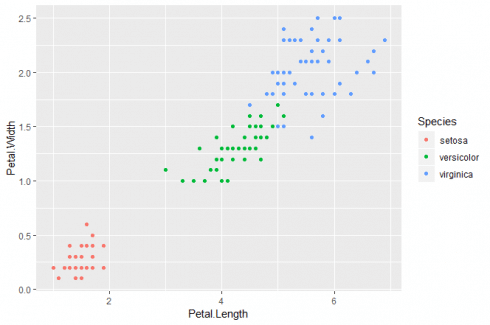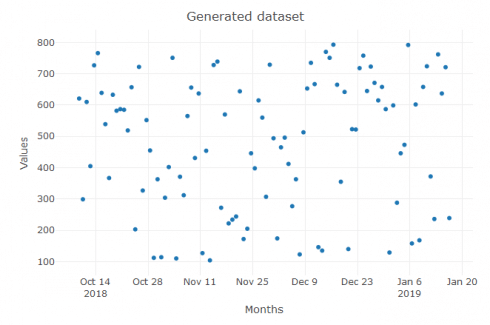October 10, 2018
By

(This article was first published on R Programming – DataScience+, and kindly contributed to R-bloggers)

Categories

Tags

One of the most demanded skills of the data analyst/scientist in 2018 is visualization. Organizations and companies have a huge demand for data visualization because this branch of methods is very efficient for the recognition of patterns and getting insight into big data.

In this article, I show how you can add value to your visualizations by making them interactive with efficient packages in R. Get ready to utilize the power of: `ggplot2`, `dygraphs` and `plotly`!

### High quality visualizations with `ggplot2`

`ggplot2` was really a gamechanger in data science when it was realeased for R Statistical Computing in 2007. This package revolutionised the possibilities for coding high quality and elegant visualisations in data science software. The package have been optimised and updated since it was released. The below coding shows how you make different kinds of visualisations with `ggplot2`:

```# Load visualisation packages & datasets
library(ggplot2)
library(colorspace)
library(datasets)

#Scatter plot with clusters
spc<-ggplot(iris, aes(Petal.Length, Petal.Width, color = Species)) + geom_point()
spc
```

The above coding gives first a scatter cluster plot with `ggplot2`:```# line plot
lpc<-ggplot(iris, aes(x=Petal.Width, y=Petal.Length, group=Species)) +
geom_line(aes(color=Species))+
geom_point(aes(color=Species))
lpc```

### Elegant and interactive visualizations with `dygraphs`

Another value generating visualisation package in R is `dygraphs`. This package focuses on creating interactive visualisations with elegant interactive coding modules. Furthermore, the package specialises in creating visualisations for machine learning methods. The below coding generates different visualisation graphs with `dygraphs`:

```# Data management packages in R
library(zoo)
library(xts)
# dygraphs in R
library(dygraphs)
# Generate dataset
value<-sample(100:800,100,replace = T)
time<-seq(from=Sys.time(),to=Sys.time()+60*60*24*99,by="day")
data<-data.frame(time=time,value=value)

# dygraph lineplot
dygraph(dy_data)
```
```# dygraph stepplot
dygraph(dy_data)  %>% dyOptions(colors="red", pointSize = 2,drawGrid =T,stepPlot = T) %>% dyRangeSelector()
```

Therafter it also creates the following interactive stepplot:### Value-adding and interactive graphs with `plotly`

The last package in this article about value adding visualisation packages in R is `plotly`. This package focuses on creating interactive and value-adding visualisations and has a clear design as well. The below coding creates different visualisations in `plotly`:

```#plotly graphs
library(plotly)
# Data management packages in R
library(zoo)
library(xts)
# Generate dataset
value<-sample(100:800,100,replace = T)
time<-seq(from=Sys.time(),to=Sys.time()+60*60*24*99,by="day")
data<-data.frame(time=time,value=value)

#plotly lineplot
lp % add_trace(y = value,mode = 'lines')%>%
layout(title = "Generated dataset",
xaxis = list(title = "Months"),
yaxis = list (title = "Values"))
lp
```
```#plotly line marker plot
lm% add_trace(y = value,mode = 'lines+markers')%>%
layout(title = "Generated dataset",
xaxis = list(title = "Months"),
yaxis = list (title = "Values"))
lm
```

The new coding gives us an interactive line marker plot in `plotly`:```#plotly marker plot
m% add_trace(y = value,mode = 'markers')%>%
layout(title = "Generated dataset",
xaxis = list(title = "Months"),
yaxis = list (title = "Values"))
m
```

The 3rd coding gives us the following interactive marker plot in `plotly`:### References

Related Post

R-bloggers.com offers daily e-mail updates about R news and tutorials on topics such as: Data science, Big Data, R jobs, visualization (ggplot2, Boxplots, maps, animation), programming (RStudio, Sweave, LaTeX, SQL, Eclipse, git, hadoop, Web Scraping) statistics (regression, PCA, time series, trading) and more...